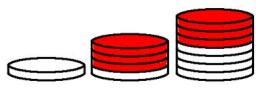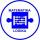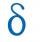# Sequence

Write the first 7 members of an arithmetic sequence: a1=-3, d=6.

a1 =  -3
a2 =  3
a3 =  9
a4 =  15
a5 =  21
a6 =  27
a7 =  33

### Step-by-step explanation:

${a}_{1}=-3$
${a}_{2}={a}_{1}+6=3$
${a}_{3}={a}_{2}+6=9$
${a}_{4}={a}_{3}+6=15$
${a}_{5}={a}_{4}+6=21$
${a}_{6}={a}_{5}+6=27$
${a}_{7}={a}_{6}+6=33$Did you find an error or inaccuracy? Feel free to write us. Thank you!## Related math problems and questions:

• Sequence 2Write the first 5 members of an arithmetic sequence a11=-14, d=-1
• SequenceWrite the first 6 members of this sequence: a1 = 5 a2 = 7 an+2 = an+1 +2 an
• Five membersWrite first 5 members geometric sequence and determine whether it is increasing or decreasing: a1 = 3 q = -2
• Sequence 3Write the first 5 members of an arithmetic sequence: a4=-35, a11=-105.
• SequenceIn the arithmetic sequence is a1=-1, d=4. Which member is equal to the number 203?
• SequenceIn the arithmetic sequence is given: Sn=2304, d=2, an=95 Calculate a1 and n.
• AP - simpleFind the first ten members of the sequence if a11 = 132, d = 3.
• Geometric sequence 4It is given geometric sequence a3 = 7 and a12 = 3. Calculate s23 (= sum of the first 23 members of the sequence).
• Sum of membersWhat is the sum of the first two members of the aritmetic progression if d = -4.3 and a3 = 7.5?
• AS - sequenceWhat are the first ten members of the sequence if a11=22, d=2.
• AP 6Calculate the first five items of an arithmetic sequence if it is given: a2 – a3 + a5 = 20 a1 + a6 = 38
• Geometric sequence 3In geometric sequence is a8 = 312500; a11= 39062500; sn=1953124. Calculate the first item a1, quotient q, and n - number of members by their sum s_n.
• Sequence - 5 membersWrite first five members of the sequence ?
• 6 termsFind the first six terms of the sequence. a1 = 7, an = an-1 + 6
• Geometric sequence 5About members of geometric sequence we know: ? ? Calculate a1 (first member) and q (common ratio or q-coefficient)
• SequenceBetween numbers 1 and 53 insert n members of the arithmetic sequence that its sum is 702.
• Difference APCalculate the difference of arithmetic progression if the sum of its first 19 members Sn = 8075 and the first member is a1 = 20